# RD Sharma Solutions for Class 11 Maths Exercise 22.2 Chapter 22 - Brief review of Cartesian System of Rectangular Coordinates

This exercise mainly deals with problems based on locus and equation to a locus. Subject experts who are highly experienced and have vast knowledge about the concepts have developed the solutions, which match the understanding ability of the students. Students can use RD Sharma Class 11 Maths Solutions as the best reference material, which consists of solutions explained in the most precise language that any student can understand. The solutions of this exercise are readily available in the pdf format, which can be downloaded easily from the links given below.

## Download the pdf of RD Sharma Solutions for Class 11 Maths Exercise 22.2 Chapter 22 – Brief review of Cartesian System of Rectangular Coordinates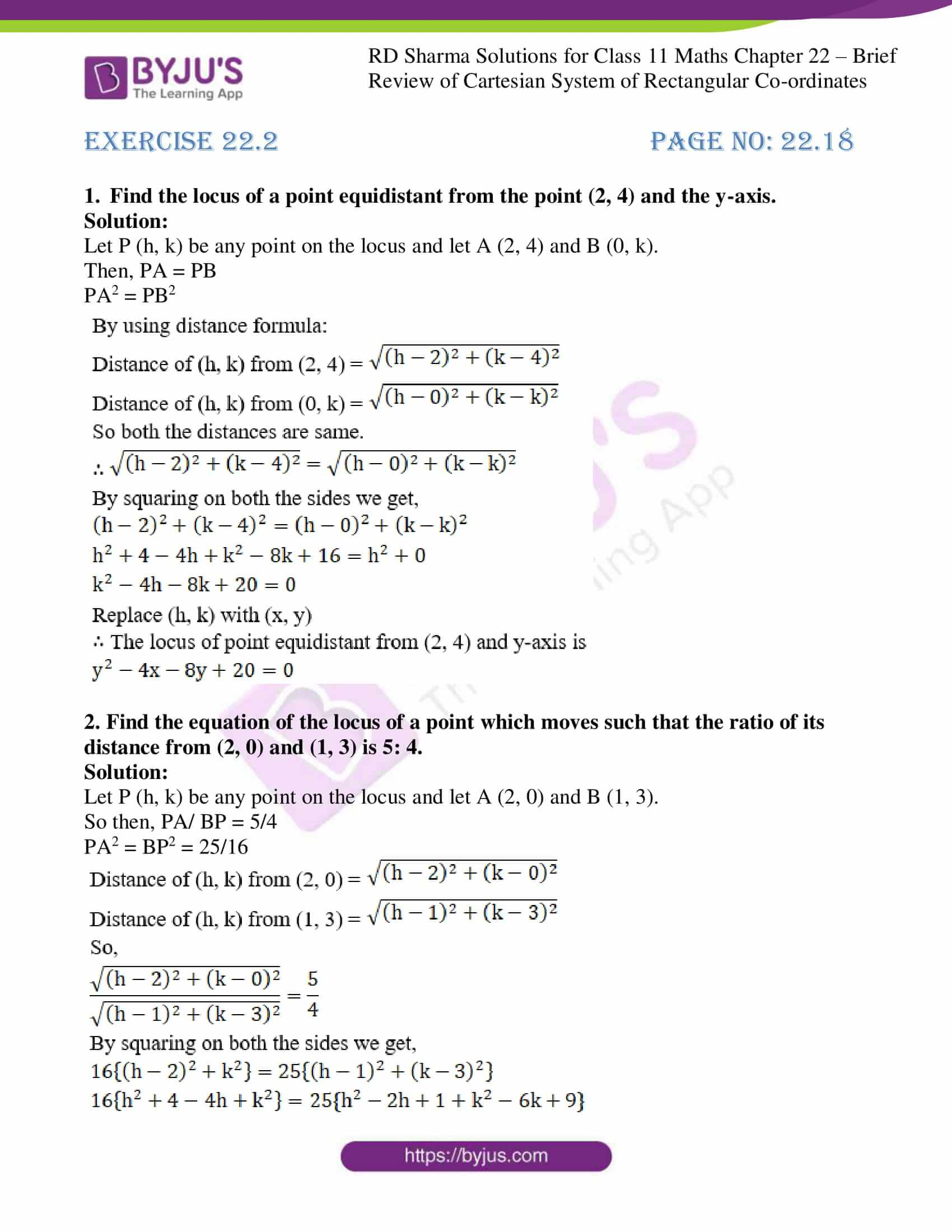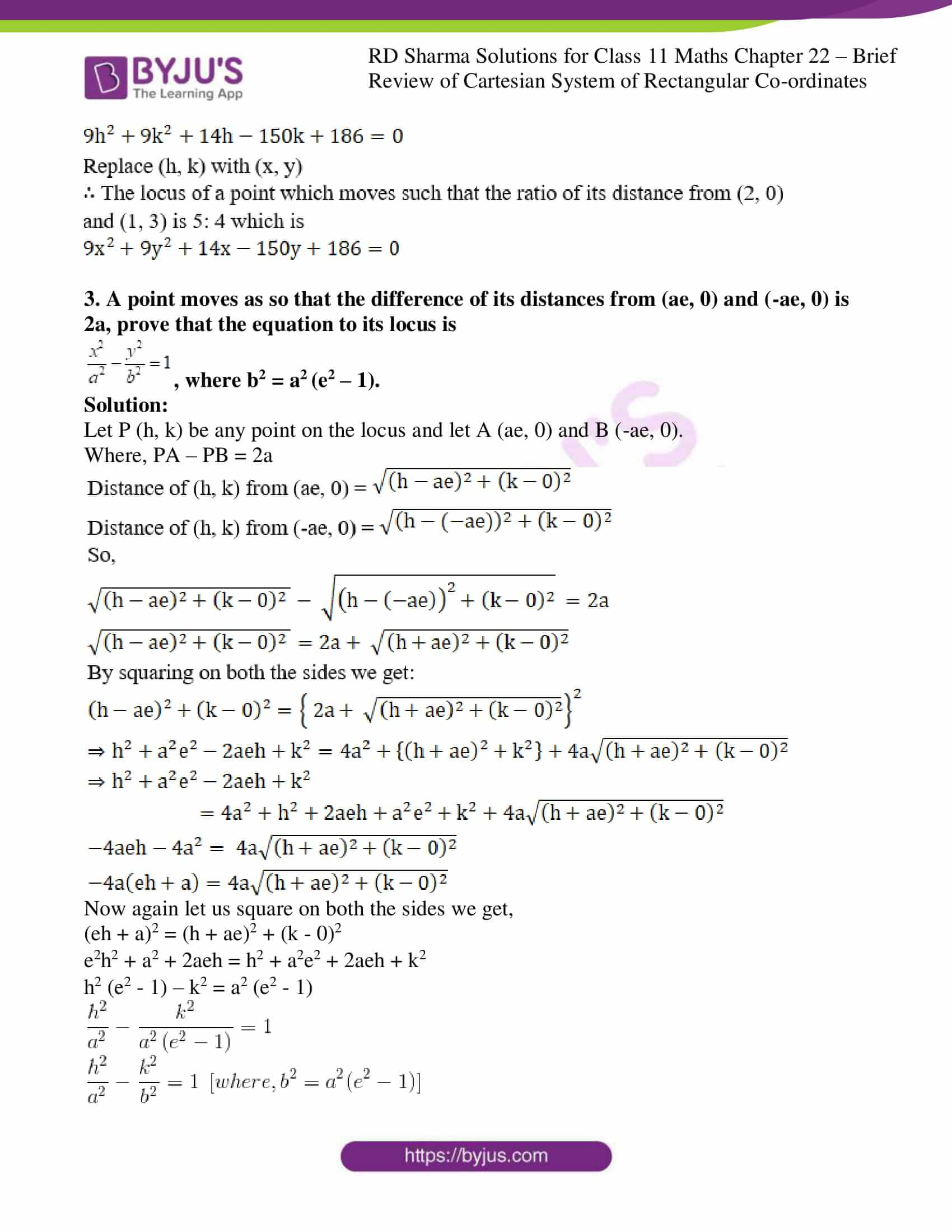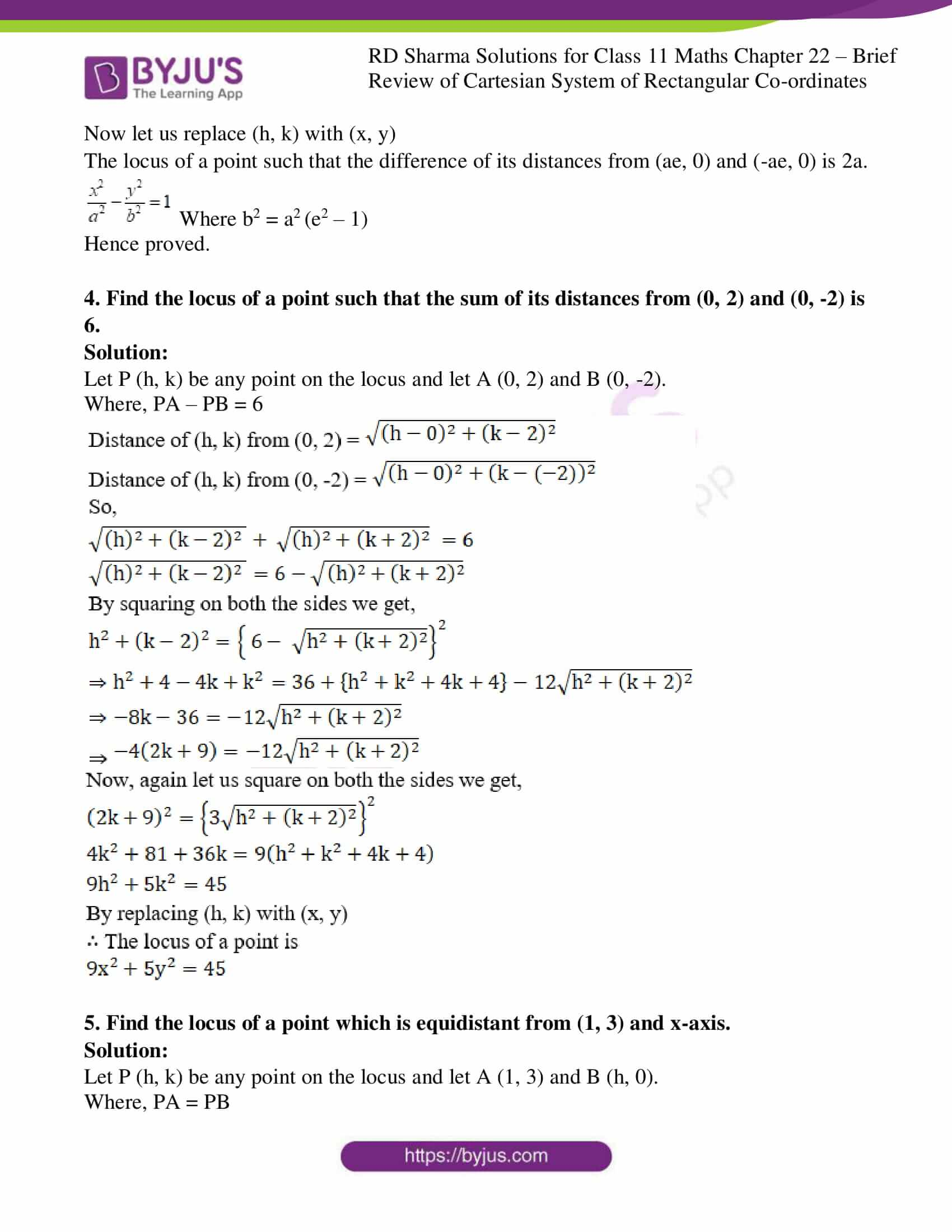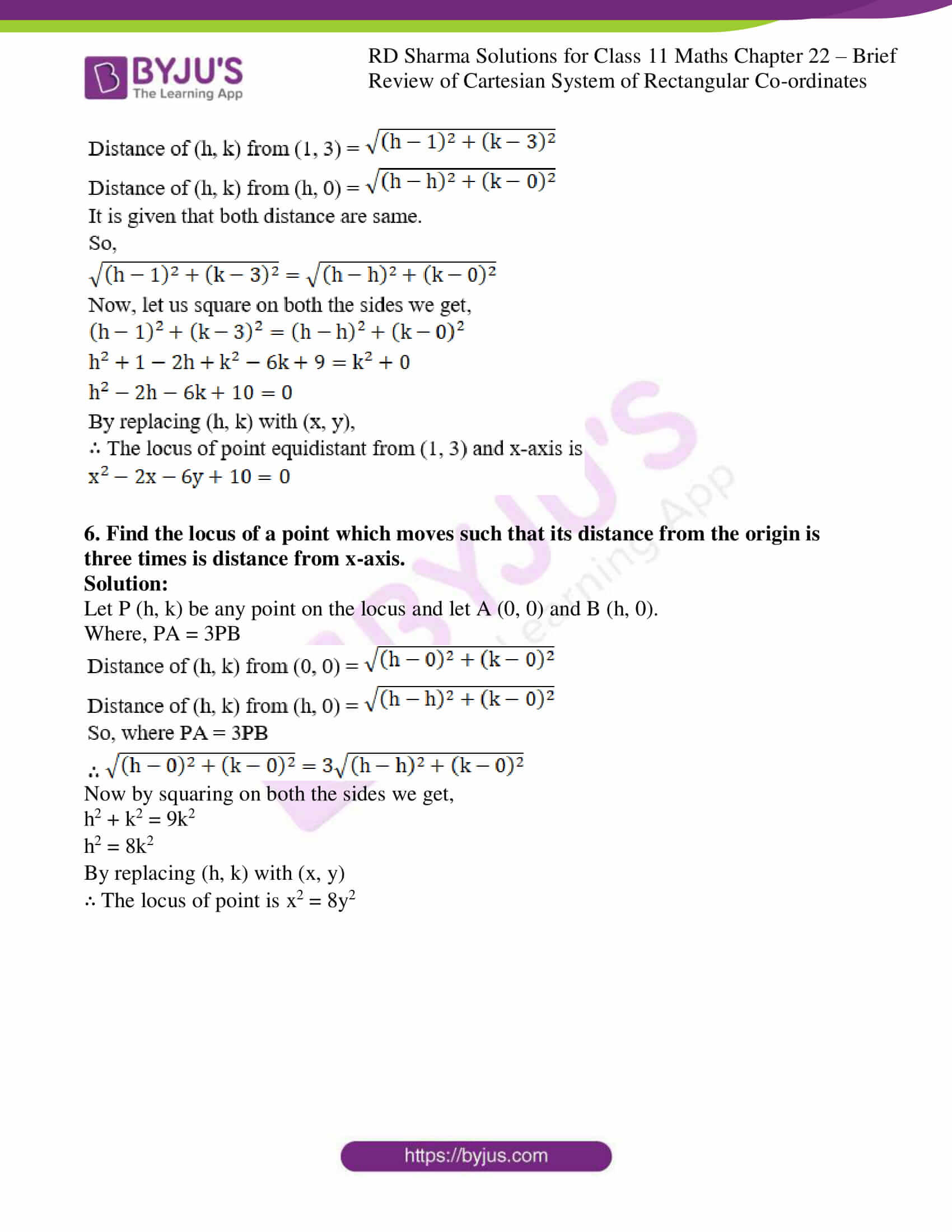### Access answers to RD Sharma Solutions for Class 11 Maths Exercise 22.2 Chapter 22 – Brief review of Cartesian System of Rectangular Coordinates

1. Find the locus of a point equidistant from the point (2, 4) and the y-axis.

Solution:

Let P (h, k) be any point on the locus and let A (2, 4) and B (0, k).

Then, PA = PB

PA2 = PB2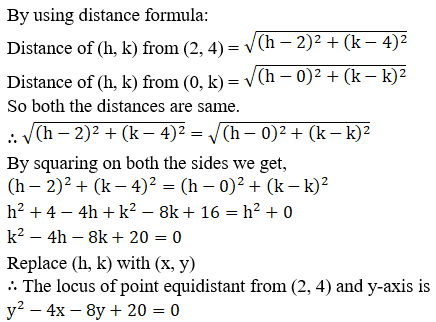2. Find the equation of the locus of a point which moves such that the ratio of its distance from (2, 0) and (1, 3) is 5: 4.

Solution:

Let P (h, k) be any point on the locus and let A (2, 0) and B (1, 3).

So then, PA/ BP = 5/4

PA2 = BP2 = 25/16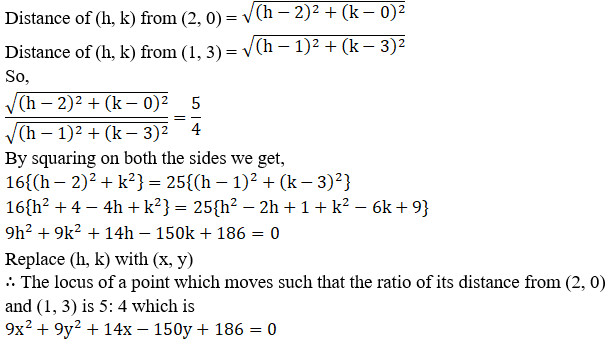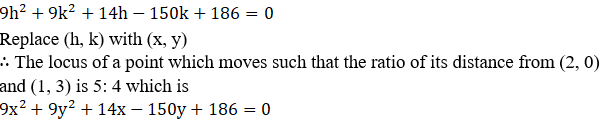3. A point moves as so that the difference of its distances from (ae, 0) and (-ae, 0) is 2a, prove that the equation to its locus is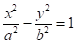, where b2 = a2 (e2 – 1).

Solution:

Let P (h, k) be any point on the locus and let A (ae, 0) and B (-ae, 0).

Where, PA – PB = 2a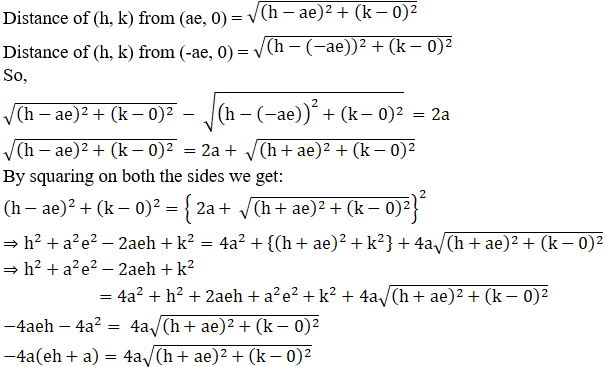Now again let us square on both the sides we get,

(eh + a)2 = (h + ae)2 + (k – 0)2

e2h2 + a2 + 2aeh = h2 + a2e2 + 2aeh + k2

h2 (e2 – 1) – k2 = a2 (e2 – 1)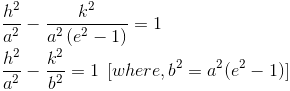Now let us replace (h, k) with (x, y)

The locus of a point such that the difference of its distances from (ae, 0) and (-ae, 0) is 2a.Where b2 = a2 (e2 – 1)

Hence proved.

4. Find the locus of a point such that the sum of its distances from (0, 2) and (0, -2) is 6.

Solution:

Let P (h, k) be any point on the locus and let A (0, 2) and B (0, -2).

Where, PA – PB = 6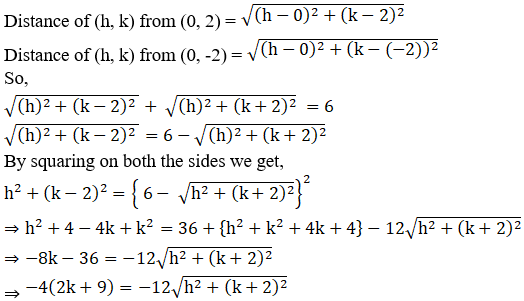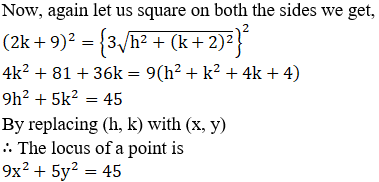5. Find the locus of a point which is equidistant from (1, 3) and x-axis.

Solution:

Let P (h, k) be any point on the locus and let A (1, 3) and B (h, 0).

Where, PA = PB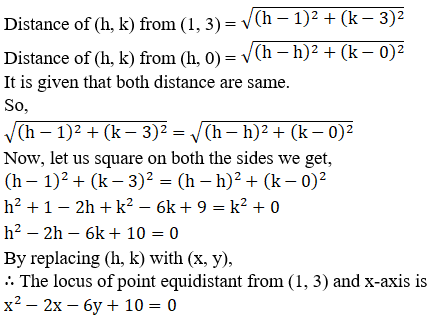6. Find the locus of a point which moves such that its distance from the origin is three times is distance from x-axis.

Solution:

Let P (h, k) be any point on the locus and let A (0, 0) and B (h, 0).

Where, PA = 3PB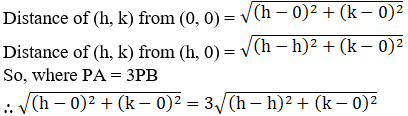Now by squaring on both the sides we get,

h2 + k2 = 9k2

h2 = 8k2

By replacing (h, k) with (x, y)

∴ The locus of point is x2 = 8y2